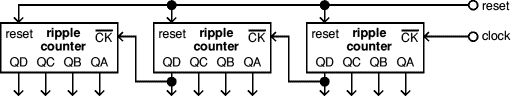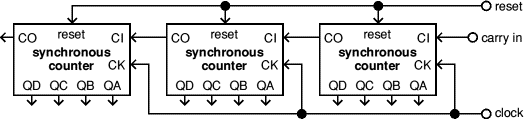# Counting Circuits

Next Page: Quantities and Units

Also See: 4000 series | 74 series | Gates

## Binary numbers

Electronic circuits count in binary. This is the simplest possible counting system because it uses just two digits, 0 and 1, exactly like logic signals where 0 represents false and 1 represents true. The terms low and high are also used for 0 and 1.

Counting one, two, three, four, five in binary: 1, 10, 11, 100, 101.

Binary numbers rapidly become very long as the count increases and this makes them difficult for us to read at a glance. Fortunately it is rarely necessary to read more than 4 binary digits at a time in counting circuits.

In a binary number each digit represents a multiple of two (1, 2, 4, 8, 16 etc), in the same way that each digit in decimal represents a multiple of ten (1, 10, 100, 1000 etc).

For example 10110110 in binary equals 182 in decimal:

 Bit value: 128 64 32 16 8 4 2 1 Binary: 1 0 1 1 0 1 1 0 Decimal: 128 +0 +32 +16 +0 +4 +2 +0 = 182

#### Seen on a T-shirt:

There are 10 kinds of people:
those who understand binary,
and those who don't.

## Bits, bytes and nibbles

Each binary digit is called a bit, so 10110110 is an 8-bit number.

A block of 8 bits is called a byte and it can hold a maximum number of 11111111 = 255 in decimal.

Computers and PIC microcontrollers work with blocks of 8 bits. Two (or more) bytes make a word, for example PICs work with a 16-bit word (two bytes) which can hold a maximum number of 65535.

A block of 4 bits is called a nibble (half a byte!) and it can hold a maximum number of 1111 = 15 in decimal.

Many counting circuits work with blocks of 4 bits because this number of bits is required to count up to 9 in decimal. (The maximum number with 3 bits is only 7).

Hexadecimal (often just called 'hex') is base 16 counting with 16 digits. It starts with the decimal digits 0-9, then continues with letters A (10), B (11), C (12), D (13), E (14) and F (15). Each hexadecimal digit is equivalent to 4 binary digits, making conversion between the two systems relatively easy.

You may find hexadecimal used with PICs and computer systems but it is not generally used in simple counting circuits.

#### Example:

10110110 binary = B6 hexadecimal = 182 decimal.

## 4-bit Numbers & Binary Coded Decimal (BCD)

The table shows the 4-bit numbers with their decimal and hexadecimal values.

The labels A,B,C,D are widely used in electronics to represent the four bits:

• A = 1, the 'least significant bit' (LSB)
• B = 2
• C = 4
• D = 8, the 'most significant bit' (MSB)

Binary Coded Decimal (BCD) is a special version of 4-bit binary where the count resets to zero (0000) after the ninth count (1001). It is used by decade counters and is easily converted to display the decimal digits 0-9 on a 7-segment display.

Several decade counters using BCD can be linked together to separately count the decimal ones, tens, hundreds, and so on. This is much easier than attempting to convert large binary numbers (such as 10110110) to display their decimal value.

Do not confuse BCD which stands for Binary Coded Decimal with the labels A,B,C,D used to represent the four binary digits - it is an unfortunate coincidence that the letters BCD occur in both!

 BinaryD C B A Decimalbase 10 Hexadecimalbase 16 0 0 0 00 0 0 10 0 1 00 0 1 10 1 0 00 1 0 10 1 1 00 1 1 11 0 0 01 0 0 11 0 1 01 0 1 11 1 0 01 1 0 11 1 1 01 1 1 1 0123456789101112131415 0123456789ABCDEF

## Counters

All counters require a 'square wave' clock signal to make them count. This is a digital waveform with sharp transitions between low (0V) and high (+Vs), such as the output from a 555 astable circuit.

Most switches bounce when the contacts close giving a rapid series of pulses. Connecting a switch directly to a clock input will usually give several counts when the switch is operated once! One way to 'debounce' the switch is to make it trigger a 555 monostable circuit with a short time period (such as 0.1s) and use the monostable output to drive the clock input.A square wave clock signal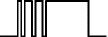The bouncing output from a switch

The animated block diagram shows a clock signal driving a 4-bit (0-15) counter with LEDs connected to show the state of the clock and counter outputs QA-QD (Q indicates an output).

The LED on the first output QA flashes at half the frequency of the clock LED. In fact the frequency of each stage of the counter is half the frequency of the previous stage. You can see this pattern too in the table above showing the 4-bit numbers.

Notice how output QA changes state every time the clock input changes from high to low (that is when the clock LED turns off), this is called the falling-edge.

Watch the counting closely and you will see that QB changes on the falling-edge of QA, QC on the falling-edge of QB and so on.

Books covering counter ICs: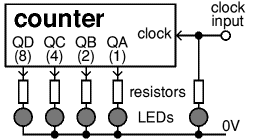A 4-bit counter and clock input
In this example counting advances on
the falling-edge of the clock signal

LED on = 1   LED off = 0

#### Breaking left to right convention!

Electronics diagram are normally drawn with inputs on the left and signals flowing to the right. This one is deliberately drawn the other way round so the outputs are placed in the correct binary order.

## Ripple and synchronous counters

There are two main types of counter: ripple and synchronous. In simple circuits their behaviour appears almost identical, but their internal structure is very different.

A ripple counter contains a chain of flip-flops with the output of each one feeding the input of the next. A flip-flop output changes state every time the input changes from high to low (on the falling-edge). This simple arrangement works well, but there is a slight delay as the effect of the clock 'ripples' through the chain of flip-flops.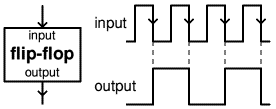The operation of a flip-flop
Notice how the output frequency
is half the input frequency

In most circuits the ripple delay is not a problem because it is far too short to be seen on a display. However, a logic system connected to ripple counter outputs will briefly see false counts which produce 'glitches' in the logic system and may disrupt its operation. For example a ripple counter changing from 0111 (7) to 1000 (8) will very briefly show 0110, 0100 and 0000 before 1000!

A synchronous counter has a more complex internal structure to ensure that all its outputs change precisely together on each clock pulse, avoiding the brief false counts which occur with ripple counters.

### Rising-edge and falling-edge clock inputs

Counting occurs when the clock input changes state.

Most synchronous counters count on the rising-edge which is the low to high transition of the clock signal.

Most ripple counters count on the falling-edge which is the high to low transition of the clock signal.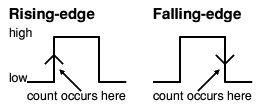It may seem odd that ripple counters use the falling-edge, but in fact this makes it easy to link counters because the most significant bit (MSB) of one counter can drive the clock input of the next. This works because the next bit must change state when the previous bit changes from high to low - the point at which a carry must occur to the next bit. Synchronous counters usually have carry out and carry in pins for linking counters without introducing any ripple delays.

## Resetting a counter

Counters can be reset to zero before their maximum count by connecting one (or more) of their outputs to their reset input, using an AND gate to combine outputs if necessary.

If the reset input is 'active-low' a NOT or NAND gate will be required to produce a low output at the desired count. If you see a line drawn above reset it means it is active low, for example: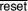(say 'reset-bar').

The reset function normally occurs immediately and you should reset on the next count above the maximum you require. For example to count 0-5 (0000-0101) you should reset on 6 (0110).

Some synchronous counters have a synchronous reset which occurs on the next clock pulse rather than immediately. This is important because you must reset on the maximum count you require. For example to count 0-5 (0000-0101), reset on 5 (0101).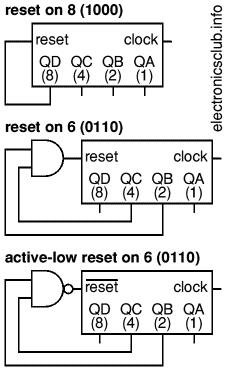### Presetting

Some counters can be preset by presenting a number to their inputs A-D and activating a preset input to load the number into the counter. By making inputs A-D all low you can also use this to reset the counter to zero.

## Frequency division

Counters can be used to reduce the frequency of an input (clock) signal. Each stage of a counter halves the frequency, so for a 4-bit (0-15) counter QA is 1/2, QB is 1/4, QC is 1/8 and QD is 1/16 of the clock frequency. Division by numbers that are not powers of 2 is possible by resetting counters.

Frequency division is one of the main purposes of counters with more than 4 bits and their outputs are usually labelled Q1, Q2 and so on. Qn is the nth stage of the counter, representing 2n. For example Q4 is 24 = 16 (1/16 of clock frequency) and Q12 is 212 = 4096 (1/4096 of clock frequency).

## Decoders

The most popular type is a 1-of-10 decoder which contains a network of logic gates to make one of its ten outputs Q0-9 become high (or low) in response to the BCD (binary coded decimal) inputs A-D. For example an input of binary 0101 (=5) will activate output Q5.

Decoders can be used for a simple counting display and for switching LEDs in sequences. The outputs must never be directly connected together, but diodes can be used to combine them as shown in the diagram.

For example using diodes to combine the 2nd (Q1) and 4th (Q3) outputs will make an LED flash twice followed by a longer gap. The top diagram shows this for a decoder where the outputs become low when activated and the bottom diagram for a decoder where the outputs become high when activated.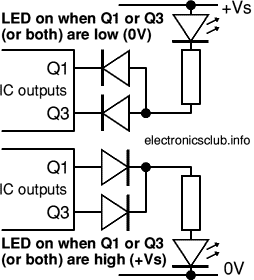## 7-segment display drivers

The inputs A-D of a display driver are connected to the BCD (binary coded decimal) outputs QA-D from a decade counter. A network of logic gates inside the display driver makes its outputs a-g become high or low as appropriate to light the required segments a-g of a 7-segment display.

A resistor is required in series with each segment to protect the LEDs, 330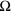is a suitable value for many displays with a 4.5V to 6V supply. Beware that these resistors are sometimes omitted from circuit diagrams!

There are two types of 7-segment displays:

• Common Anode (CA or SA) with all the LED anodes connected together. These need a display driver with outputs which become low to light each segment. Connect the common anode to +Vs.
• Common Cathode (CC or SC) with all the cathodes connected together. These need a display driver with outputs which become high to light each segment. Connect the common cathode to 0V.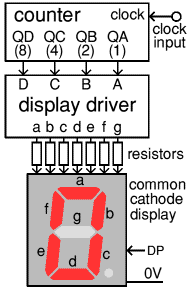driver and 7-segment display

The common anode/cathode is often available on 2 pins. Displays also have a decimal point (DP) but this is not controlled by the display driver. The segments of larger displays have two LEDs in series. For display connections please see your supplier's website or catalogue, or the manufacturer's datasheet.

### Multiplexing

If there are many 7-segment display digits multiplexing is usually used. This is a system of switching so that of all the decade counters share a single display driver which is connected to all of the displays. The output of each counter is connected in turn to the inputs of the display driver and at the same time the common anode/cathode of the corresponding 7-segment display is connected so that only one display lights at a time.The switching is done very rapidly (typically 400 - 1000Hz) and the segment current is larger than normal so the display appears continuous and of normal brightness. Multiplexing requires ICs to do the switching, but the complete circuit has fewer ICs than having one display driver for each display.

Counters may be linked together in a chain to count larger numbers. It may seem tempting to use a 12-bit or 14-bit counter, but it is not practical to convert their large binary numbers to decimal. You should use a chain of decade (0-9) counters which use BCD (binary coded decimal) to make the conversion to decimal very easy: the first counts the units, the second counts the tens, the third the hundreds and so on.

Some dual counter ICs are available with two separate counters on the same IC, the two counters must be linked externally if required (there is no internal link).

The way that counters are linked depends on the nature of the counter. The diagrams below show the general arrangements for standard ripple and synchronous counters but it is important to read the detailed information for particular counters, consulting a datasheet if necessary.

The diagram below shows how to link standard ripple counters. Notice how the highest output QD of each counter drives the clock (CK) input of the next counter. This works because ripple counters have clock inputs that are 'active-low' which means that the count advances as the clock input becomes low, on the falling-edge.

Remember that with all ripple counters there will be a slight delay before the later outputs respond to the clock signal, especially with a long counter chain. This is not a problem in simple circuits driving displays, but it may cause glitches in logic systems connected to the counter outputs.